# Mathematics Reference

The Diigo Reference list (see I’m Looking For…page for this and other lists) includes many useful resources including Mathematics dictionaries.

Note that these resources are also given on my blog written for students.

Two particularly useful dictionaries for students of all ages are those from Jenny Eather
(Note the excellent section on charts also).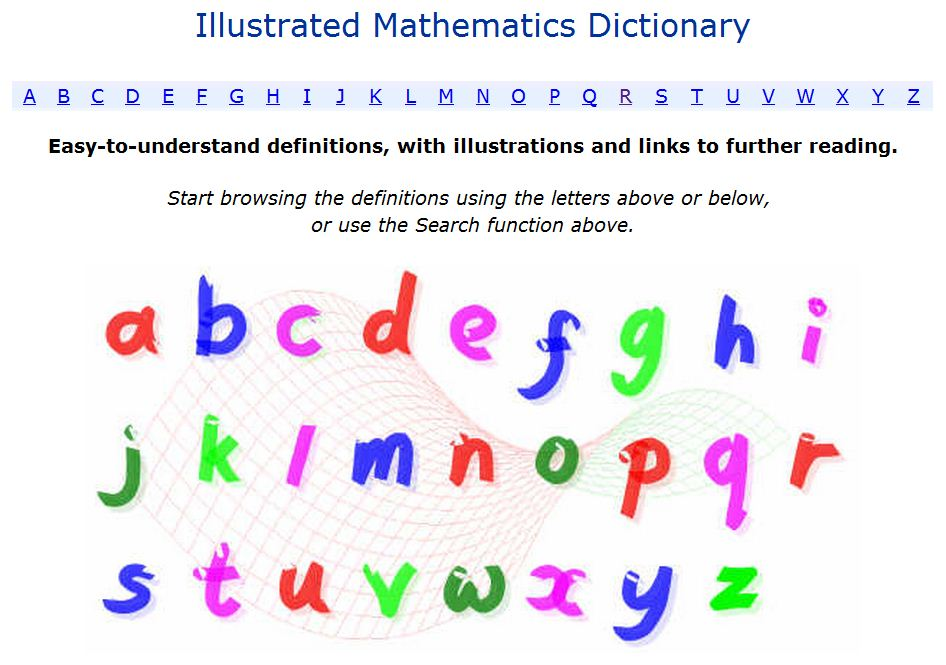The Diigo Reference list also includes sites on the history of  Mathematics.

Checking the University of St Andrews site we can find out about mathematicians who were born or died on this day.

# Order of Operations

There are several resources to help demonstrate the correct order of operations in a calculation.

From the always relaible CIMT, one of their interactive sections gives examples and exercises.

..or try this game (requires Flash) from Colleen King’s Math Playground. Use number tiles to create a sum with the answer given.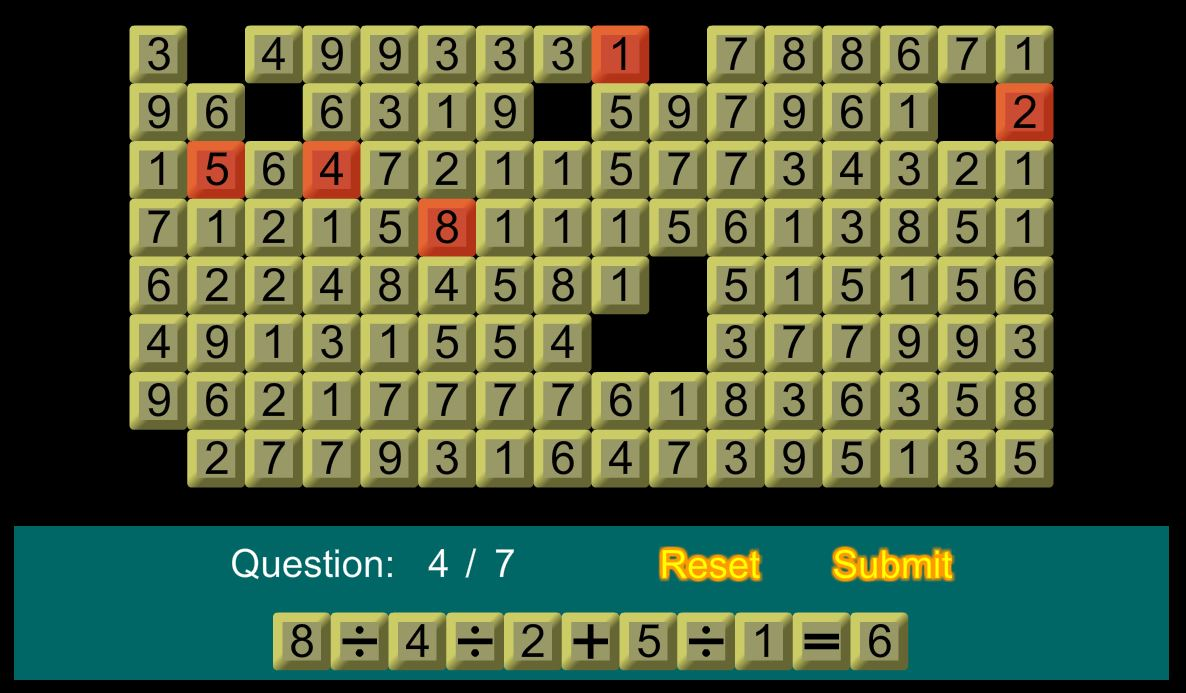and of course, you can always check answers on WolframAlpha!

By Colleen Young Posted in Number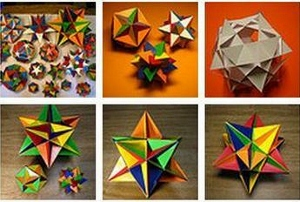# Mathematical Images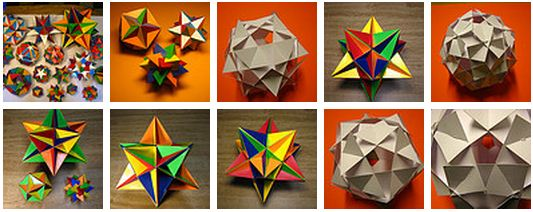(Images returned from a Wylio search on polyhedra).

Do you or your students ever need images? Care should be taken with copyright when using images from the Internet.

There are many sources of copyright free images. The above images (and many more) were returned as a result of searching Wylio for polyhedron. This Diigo list (clicking on the green links takes you direct to each website) has several sites which can be searched for copyright free images.

Note the end of the list has some sites specific to Mathematics, these are not necessarily copyright free (except those from the Pics4Learning site) but there are many interesting images to show students such as those from the MAA’s ‘Found Math’ project.

See this post for information for students.

And of course you can always take your own photos! More holiday pictures!# Solving Equations

A collection of resources to use to demonstrate and practise solving equations.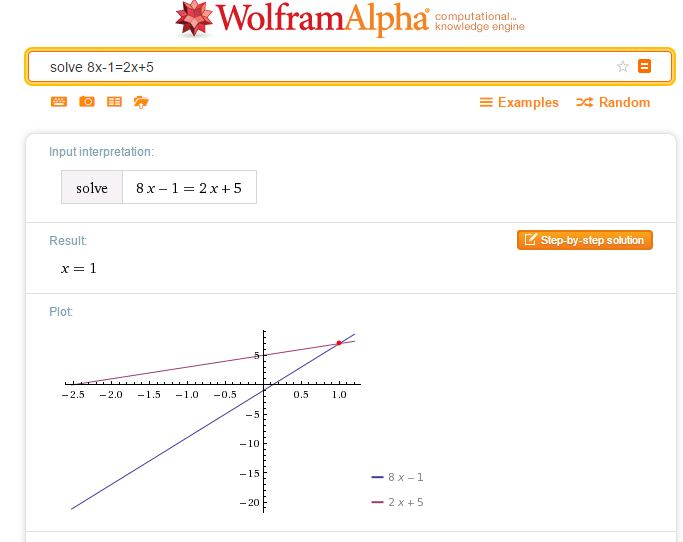A simple way to check a solution to an equation of any type is to simply enter your equation as a WolframAlpha query. Note that WolframAlpha includes a graphical illustration; it is so important for students to understand how equations may be solved graphically; I always illustrate graphical solutions when we are working with Algebra to help students make these links. Desmos of course, is ideal here.The following resources allow practice in choosing the correct operations to reach a solution.

Centre for Innovation in Mathematics Teaching
CIMT
have tutorials on equations: Linear Equations 1, Linear Equations 2, and Linear Equations with Brackets in their Interactive ResourcesChoose the type of equation you require then the sequence of operations required to solve the equation.
Select Do it after each operation, for example -32 Do it were the keys selected to start the above problem.

The next sites work in a similar way to the subtangent resource.

Flashy MathsSolving Equations (select the swf file to play online)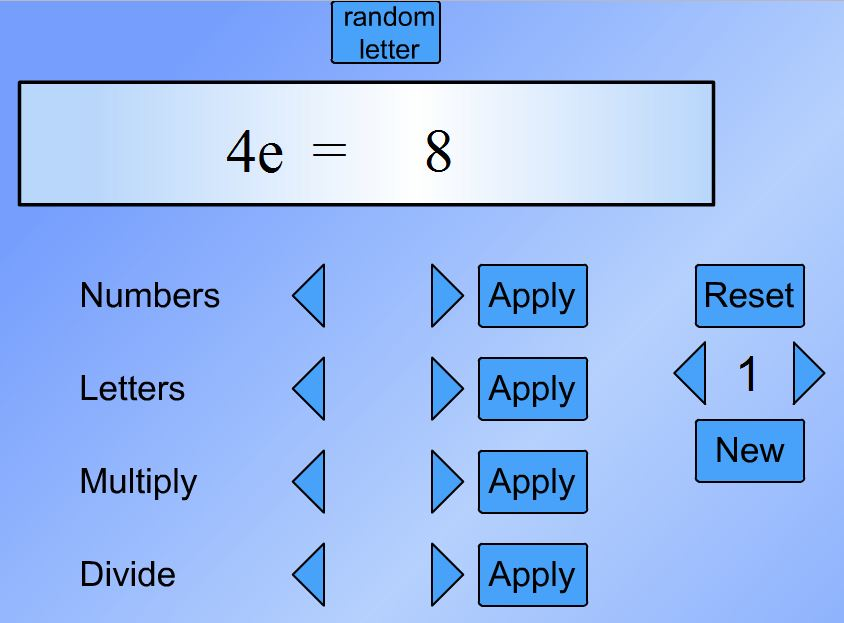There are 4 levels to choose from: the level 1 equations are of the type ax = b,
level 2, x±c = d, level 3, ex±f = g and level 4, hx±i = hx±j = k.
Choose the series of operations you require to solve the equation selecting apply at each stage to see the result of your chosen operation.
Choose numbers to add and subtract integers and letters to add and subtract variables.

MathsNetEquation Buster
Playing at level 5 introduces equations with fractions.From the National Library of Virtual Manipulatives try Algebra Balance Scales and Algebra Balance Scales – Negatives.
Note that these manipulatives allow you to create a problem of your own (so long as it has an integer solution) as well as solving problems given.From WaldoMaths, try this applet on solving simple linear equations and this on equations requiring more complex manipulation.
Each applet has several levels.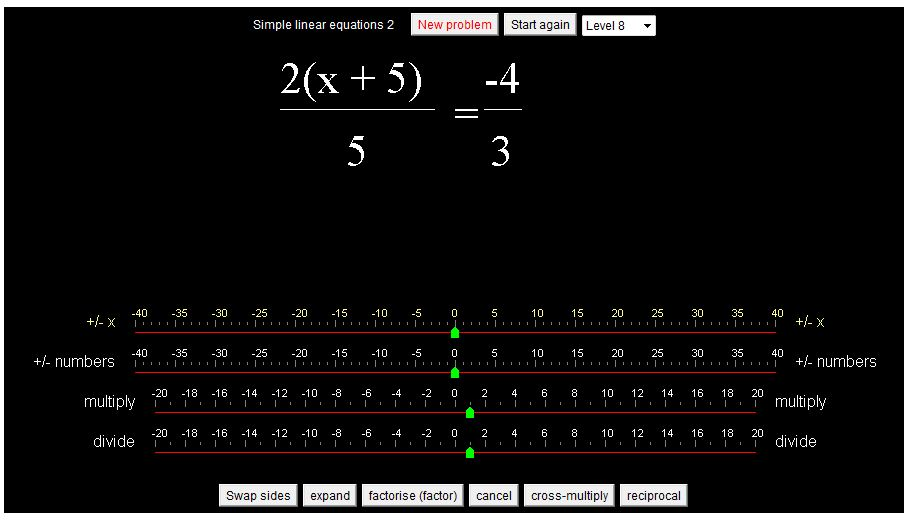Unlike the other resources this allows the user to enter the equation resulting from the chosen operation.
The solution is checked at each stage.
As well as solving the given problems (20 problems) the user can select ‘Make an equation yourself’ to enter any equation then solve that equation.
(Unlike the NLVM resource above the x can be any real number).

The following video shows the keystrokes required to enter and solve an equation.